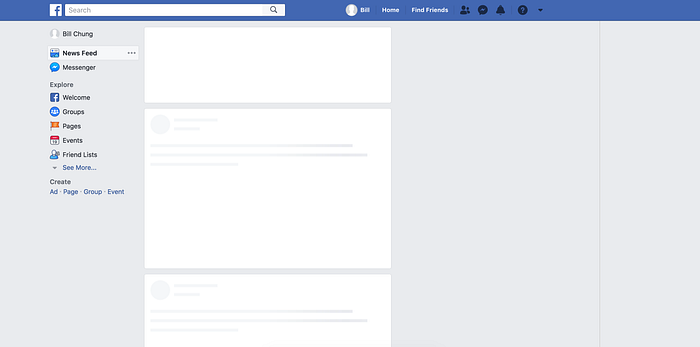Wanna see something cool? Check out Angular Spotify 🎧

Mode Description
determinate Standard progress indicator fills from 0% to 100%
indeterminate Indicates that something is happening without conveying discrete progress

Spinners and progress bars are explicit loading paradigms. They focus the user on communicating a loading period and, more often than not, are blocking user interaction until a layout has loaded enough to be useful.

No one wants to wait 🤣

Introducing placeholder loading, it is usually grey or neutral-toned filled shapes, meet the user instantly upon user interaction with calls to action or links. The placeholders (the so-called “bones” of the skeleton) are then replaced with the actual site content, and the illusion is complete. That’s what skeleton screens do: create the illusion of an instant transition.

A famous example is Facebook’ newsfeed.Based on a research, displaying a skeleton screen will cause humans to perceive a loading period as being shorter in duration

On jira.trungk18.com, I also need a placeholder loading for an issue detail. I could display a spinner, but too much spinner doesn’t seem like an excellent way to interact with your user. 😂

The standard-issue detail will have two columns layout.

I translated the above detail layout to gray `rect` and `circle` SVG at the end.I used ngneat/content-loader for this purpose. You need to prepare an SVG structure, and `content-loader` will do the rest of the work for you, including the animation.

### Install

``yarn add @ngneat/content-loader``

### Usage

``````import { ContentLoaderModule } from '@ngneat/content-loader'

@NgModule({
})
export class AppModule {}``````

``````<content-loader>
<svg:rect x="0" y="0" rx="3" ry="3" width="250" height="10" />
<svg:rect x="20" y="20" rx="3" ry="3" width="220" height="10" />
<svg:rect x="20" y="40" rx="3" ry="3" width="170" height="10" />
<svg:rect x="0" y="60" rx="3" ry="3" width="250" height="10" />
<svg:rect x="20" y="80" rx="3" ry="3" width="200" height="10" />
<svg:rect x="20" y="100" rx="3" ry="3" width="80" height="10" />

Warning: Safari renders the SVG in black in case your Angular application uses the `<base href="/"/>` tag in the `<head/>` of your `index.html`. Refer to the input property `baseUrl` on the library documentation to fix this issue.

### What is svg:rect

There are several basic shapes used for most SVG drawing. SVG has some predefined shape elements that can be used by developers:

• Rectangle `<rect>`
• Circle `<circle>`
• Ellipse `<ellipse>`
• Line `<line>`
• Polyline `<polyline>`
• Polygon `<polygon>`
• Path `<path>`

For Angular to work, you need to specify `svg:rect` instead of purely `rect`. If you use `rect` only, it will give you an error

### Explanation

In detail for `rect`, it is a basic SVG shape that draws rectangles, defined by their `position`, `width`, and `height`. The rectangles may have their `corners rounded`.

``<svg:rect x="20" y="100" rx="3" ry="3" width="80" height="10" />``
Attribute Description Explain
x left position of the rectangle `x="20"` places the rectangle `20px` from the left margin
y top position of the rectangle `y="100"` places the rectangle `100px` from the top margin
width & height height and the width of the rectangle `width="80"` mean 80px wide and `height="10"` mean 10px tall
rx radius on the `x-axis` (horizontal corner) `rx="3"` mean 3px radius on the x-axis
ry radius on the `y-axis` (vertical corner) `ry="3"` mean 3px radius on the y-axis

Pretty straight forward, based on the issue detail layout with two columns. I went ahead and wrote the following code. You’re all set!

``````<content-loader
[viewBox]="'0 0 940 260'"
[backgroundColor]="'#f3f3f3'"
[foregroundColor]="'#ecebeb'"
>
<svg:rect x="0" y="0" rx="3" ry="3" width="627" height="24" />
<svg:rect x="0" y="29" rx="3" ry="3" width="506" height="24" />
<svg:rect x="0" y="77" rx="3" ry="3" width="590" height="16" />
<svg:rect x="0" y="100" rx="3" ry="3" width="627" height="16" />
<svg:rect x="0" y="123" rx="3" ry="3" width="480" height="16" />
<svg:rect x="0" y="187" rx="3" ry="3" width="370" height="16" />
<svg:circle cx="18" cy="239" r="18" />
<svg:rect x="46" y="217" rx="3" ry="3" width="548" height="42" />
<svg:rect x="683" y="3" rx="3" ry="3" width="135" height="14" />
<svg:rect x="683" y="33" rx="3" ry="3" width="251" height="24" />
<svg:rect x="683" y="90" rx="3" ry="3" width="135" height="14" />
<svg:rect x="683" y="120" rx="3" ry="3" width="251" height="24" />
<svg:rect x="683" y="177" rx="3" ry="3" width="135" height="14" />
<svg:rect x="683" y="207" rx="3" ry="3" width="251" height="24" />